Showing first {{hits.length}} results of {{hits_total}} for {{searchQueryText}}{{hits.length}} results for {{searchQueryText}}

No Search Results

Exams can be easily created in LaTeX by means of the class exam.cls. This class makes it straightforward to typeset questions, and it sets a 1in margin in all paper sizes and provides special commands to write and compute grades. This article explains how to edit with exam.cls. Contents 1 Introduction 2 Creating new questions 2.1 spacing 2.2 subparts 3 Multiple choice questions 4 Grading 4.1 points in each question 4.2 counting points and half points 4.3 bonus points 4.4 grading table 5 Other languages 6 Reference guide 7 Further reading Introduction Let's see a simple working example of the exam class: \documentclass{exam} \usepackage[utf8]{inputenc} \begin{document} \begin{center} \fbox{\fbox{\parbox{5.5in}{\centering Answer the questions in the spaces provided. If you run out of room for an answer, continue on the back of the page.}}} \end{center} \vspace{5mm} \makebox[\textwidth]{Name and section:\enspace\hrulefill} \vspace{5mm} \makebox[\textwidth]{Instructor’s name:\enspace\hrulefill} \begin{questions} \question Is it true that $$x^n + y^n = z^n$$ if $$x,y,z$$ and $$n$$ are positive integers?. Explain. \question Prove that the real part of all non-trivial zeros of the function $$\zeta(z)$$ is $$\frac{1}{2}$$ \question Compute $\int_{0}^{\infty} \frac{\sin(x)}{x}$ \end{questions}To use the exam class you must put the line \documentclass{exam} on top of your .tex file. This will enable the package's exam-related commands, and set the page format to allow margins for corrections. The syntax of the questions environment is very similar to that of the itemize and enumerate environments. Each question is typed by putting the command \question before it. The other commands in this example are not specific to the exam class, but may be useful to create a quick header for your exam.   Open an example of the exam class in Overleaf Creating new questions In the previous section, a basic example showing how to create question was presented. Questions can be further customized, and this section explains how. spacing If the students are required to answer the exam in the space provided, that space can be manually set or evenly distributed. See the example below: \begin{questions} \question Is it true that $$x^n + y^n = z^n$$ if $$x,y,z$$ and $$n$$ are positive integers?. Explain. \vspace{\stretch{1}} \question Prove that the real part of all non-trivial zeros of the function $$\zeta(z)$$ is $$\frac{1}{2}$$ \vspace{\stretch{1}} \question Compute $\int_{0}^{\infty} \frac{\sin(x)}{x}$ \vspace{\stretch{1}} \end{questions} \clearpage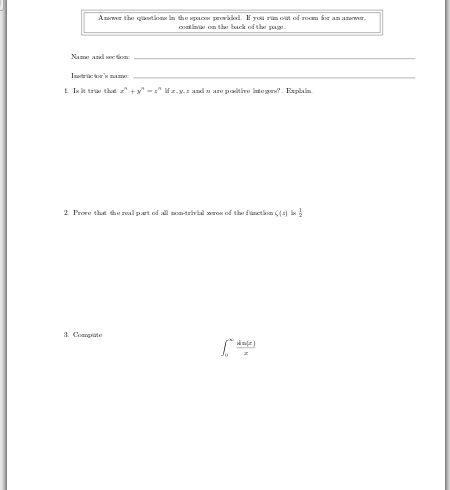In this example the command \vspace{\stretch{1}} after each question equally distributes the available space. The command \clearpage inserts a page break point to continue typing questions in a new page. If you want to manually assign the space to each question, use the command \vspace{} and in between the braces write the units of space you need. For instance, \vspace{1in} inserts a 1-inch vertical space. Check the documentation about lenghts in LaTeX for a list of available units.   Open an example of the exam class in Overleaf subparts If your questions have several parts focused on some subtopics you can use the environments parts, subparts, subsubparts and the corresponding commands \part, \subpart and \subsubpart. See the next example: \begin{questions} \question Given the equation $$x^n + y^n = z^n$$ for $$x,y,z$$ and $$n$$ positive integers. \begin{parts} \part For what values of $n$ is the statement in the previous question true? \vspace{\stretch{1}} \part For $n=2$ there's a theorem with a special name. What's that name? \vspace{\stretch{1}} \part What famous mathematician had an elegant proof for this theorem but there was not enough space in the margin to write it down? \vspace{\stretch{1}} \begin{subparts} \subpart Who actually proved the theorem? \vspace{\stretch{1}} \subpart How long did actually take to solve this problem? \vspace{\stretch{1}} \end{subparts} \end{parts} \question Prove that the real part of all non-trivial zeros of the function $$\zeta(z)$$ is $$\frac{1}{2}$$ ... \end{questions}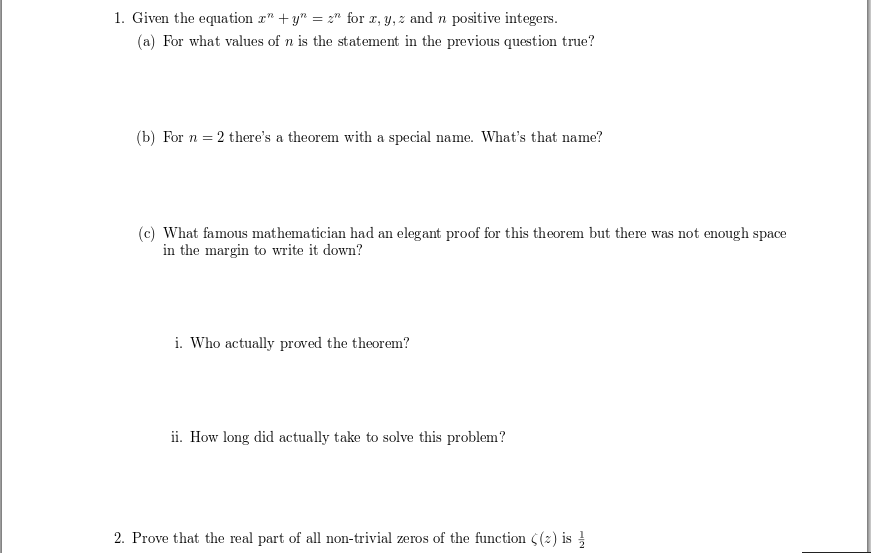The environments parts and subparts provide question-like nested lists. Jut like in questions you can set manually the vertical spacing.   Open an example of the exam class in Overleaf Multiple choice questions There are four environments to create multiple choice questions. \question Which of these famous physicists invented time? \begin{oneparchoices} \choice Stephen Hawking \choice Albert Einstein \choice Emmy Noether \choice This makes no sense \end{oneparchoices} \question Which of these famous physicists published a paper on Brownian Motion? \begin{checkboxes} \choice Stephen Hawking \choice Albert Einstein \choice Emmy Noether \choice I don't know \end{checkboxes}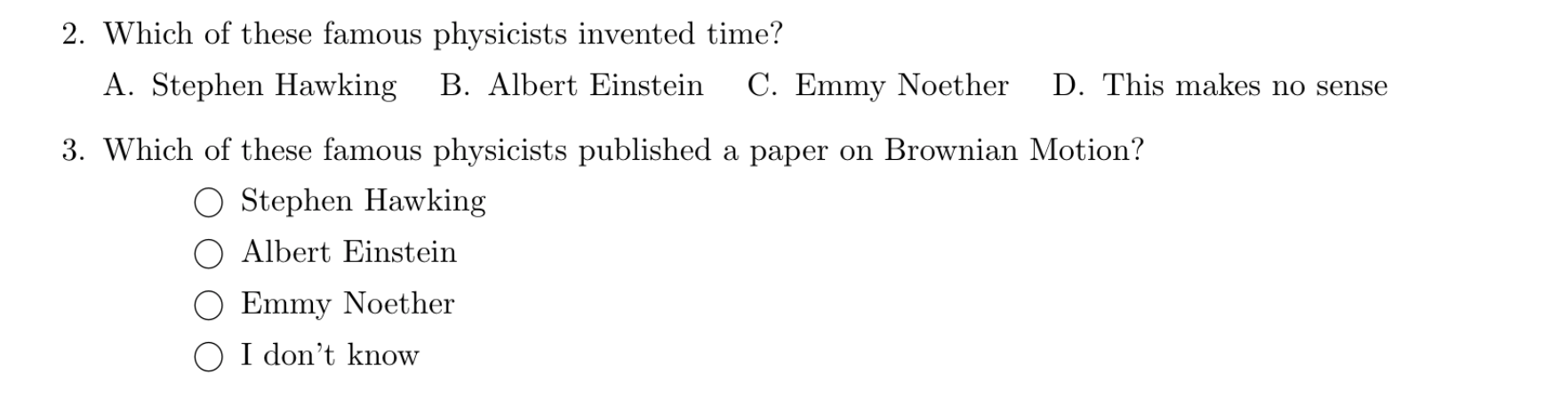In this example, two different environments are used to list the possible choices for multiple-choice questions. The environment oneparchoices labels the choices with upper case letters and prints them horizontally. If you want the choices to be printed in a list-like format, the environment choices is the right choice. The environment checkboxes prints check boxes before each choice. If you need the choices to be printed horizontally use the environment oneparcheckboxes instead.   Open an example of the exam class in Overleaf Grading Another important feature of the exam class is that it provides commands to make grading the exams easier. points in each question You can add a parameter to each \question or \part to print the number of points you attain by correctly answering it \begin{questions} \question Given the equation $$x^n + y^n = z^n$$ for $$x,y,z$$ and $$n$$ positive integers. \begin{parts} \part For what values of $n$ is the statement in the previous question true? \vspace{\stretch{1}} \part For $n=2$ there's a theorem with a special name. What's that name? \vspace{\stretch{1}} \part What famous mathematician had an elegant proof for this theorem but there was not enough space in the margin to write it down? \vspace{\stretch{1}} \end{parts} \question Compute $\int_{0}^{\infty} \frac{\sin(x)}{x}$ \vspace{\stretch{1}} \end{questions}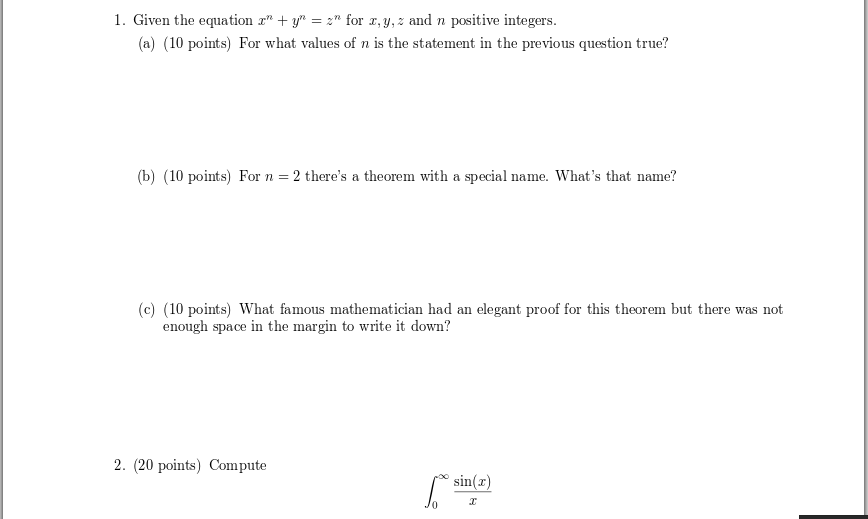The additional parameter inside brackets after a question or a part represents the number of points assigned to it. You can change the appearance and the place where the points are printed, see the reference guide for additional commands.   Open an example of the exam class in Overleaf counting points and half points Sometimes it's convenient to include half points as value for parts of a questions. You can do this and then print then the value of the whole question. See the example below: \documentclass[addpoints]{exam} \usepackage[utf8]{inputenc} \begin{document} \begin{questions} \question Given the equation $$x^n + y^n = z^n$$ for $$x,y,z$$ and $$n$$ positive integers. \begin{parts} \part For what values of $n$ is the statement in the previous question true? \vspace{\stretch{1}} \part[2 \half] For $n=2$ there's a theorem with a special name. What's that name? \vspace{\stretch{1}} \part[2 \half] What famous mathematician had an elegant proof for this theorem but there was not enough space in the margin to write it down? \vspace{\stretch{1}} \end{parts} \droptotalpoints \question... \end{questions}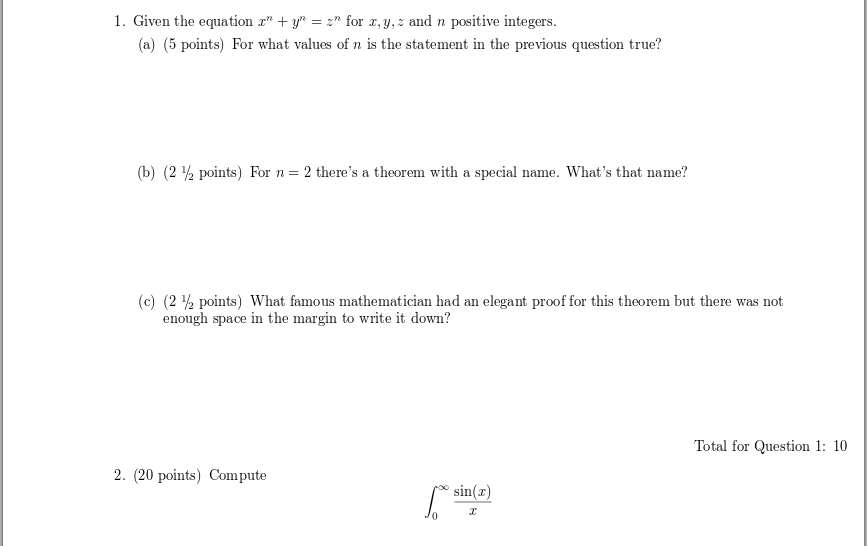The command \half adds half points to a question. The command \droptotalpoints prints the total number of points for the last question. For this last command to work you must add the option [addpoints] to the document class statement.   Open an example of the exam class in Overleaf bonus points It is possible to add bonus questions, this extra points will later show up in the grading table. Adding bonus questions and parts is actually as simple as creating regular questions and parts. \begin{questions} \question Given the equation $$x^n + y^n = z^n$$ for $$x,y,z$$ and $$n$$ positive integers. \begin{parts} \part For what values of $n$ is the statement in the previous question true? \vspace{\stretch{1}} \part[2 \half] For $n=2$ there's a theorem with a special name. What's that name? \vspace{\stretch{1}} \bonuspart[2 \half] What famous mathematician had an elegant proof for this theorem but there was not enough space in the margin to write it down? \vspace{\stretch{1}} \end{parts} \droptotalpoints \question Compute $\int_{0}^{\infty} \frac{\sin(x)}{x}$ \vspace{\stretch{1}} \bonusquestion Prove that the real part of all non-trivial zeros of the function $$\zeta(z)$$ is $$\frac{1}{2}$$ \vspace{\stretch{1}} \end{questions}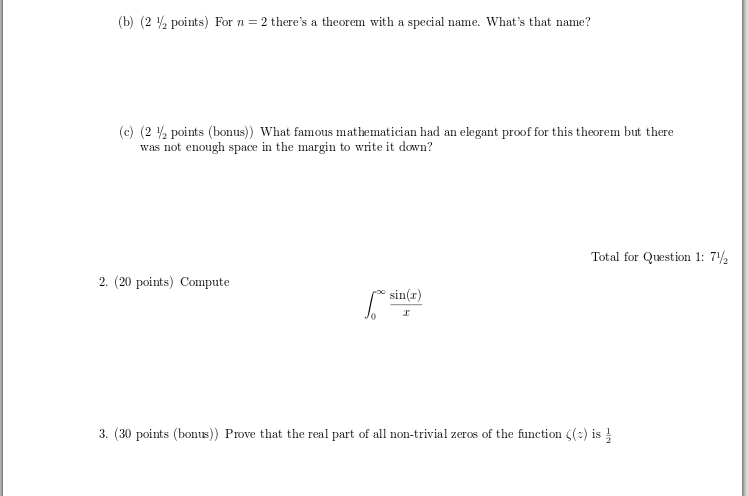The commands \bonusquestion and \bonuspart print "(bonus)" next to the point value of the question.   Open an example of the exam class in Overleaf grading table A table that show the points of each question can be printed with a special command. \begin{center} \combinedgradetable[h][questions] \end{center}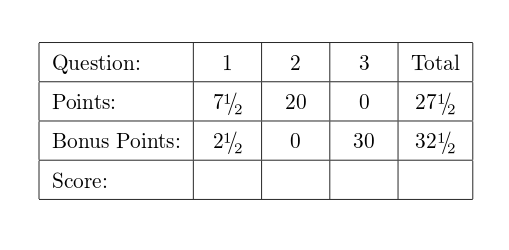There are three commands to print a table of grades: \gradetable Prints a table with the grades corresponding to regular questions. If there are bonus questions those are not included. \bonusgradetable Prints a table with the grades corresponding to the bonus questions only \combinedgradetable Prints a table with both, regular and bonus questions. These commands take two extra parameters, each parameter inside brackets. The first one can be [h] for a horizontal table or [v] for a vertical table. The second one sets how the table is indexed: [questions] to index the points by question and [pages] to list the points by page number.   Open an example of the exam class in Overleaf Other languages There is no support for other languages than English in the exam class. Nevertheless, it's easy to translate the default words for those in your local language. The next snippet shows how to translate the example presented in the previous sections to Spanish. ss[addpoints]{exam} \usepackage[utf8]{inputenc} \usepackage[spanish]{babel} \pointpoints{punto}{puntos} \bonuspointpoints{punto extra}{puntos extra} \totalformat{Pregunta \thequestion: \totalpoints puntos} \chqword{Pregunta} \chpgword{Página} \chpword{Puntos} \chbpword{Puntos extra} \chsword{Puntos obtenidos} \chtword{Total} ...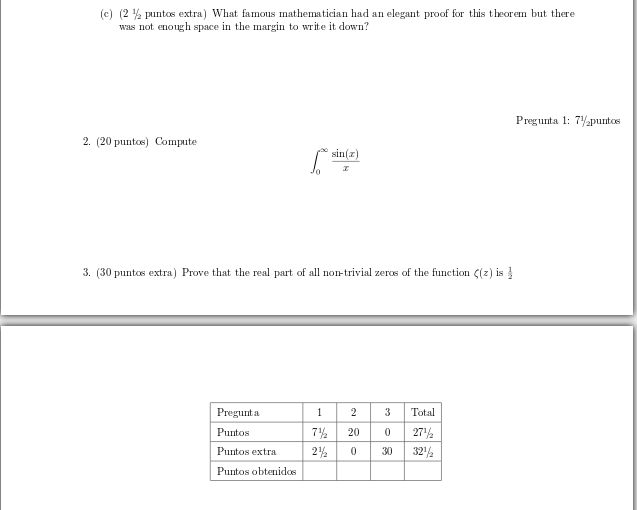The rest of the document would be exactly the same shown in previous examples. The commands typed here change the default words in the exam class. \pointpoints{punto}{puntos} Changes the words point and points for the words punto and puntos in regular questions and parts. \bonuspointpoints{punto extra}{puntos extra} Writes punto extra and puntos extras instead of bonus point and bonus points in bonus questions and bonus parts. \totalformat{Pregunta \thequestion: \totalpoints puntos} Changes the text printed by \droptotalpoints. In the example it prints Pregunta 1: 7 1/2 puntos. \chqword{Pregunta} Changes the word Question for the word Pregunta in the grading table table. The rest of the commands change the other words in that table, chpgword for Page, \chpword for Points, \chbpword for Bonus points, \chsword for Score and \chtword for Total. These commands may change depending on the type of table your are creating, see the reference guide for the commands needed in other tables.   Open an example of the exam class in Overleaf Reference guide Placing and formatting the points mark for questions These commands can be typed in the preamble to change the format of the whole document or right before a question to change the format from that question down to the next formatting command or the end of the document. \poinstmargin. The point values are printed in the left margin. Use \nopointsmargin to revert this command to the default format. \pointsmarginright. The point values are printed in the right margin. The command \nopoinstmarginright will revert to the normal behaviour. \bracketedpoints. Uses brackets instead of parentheses around the point values. \boxedpoints. Draws a box around the point values. Changing default names in Grade Tables The commands depend on the format and the information displayed on the table. The h and v within each command mean horizontal or vertical orientation. If the command is preceded by a b means it changes the format in a bonus table, if the command is preceded by a c means it works on combined tables. For instance, to change the word "Score" in a vertical oriented bonus table for the words "Points Awarded" you should use \bvsword{Points Awarded}. Below a table with the default values is shown. horizontal vertical grades table \hqword{Question:} \hpgword{Page:} \hpword{Points:} \hsword{Score:} \htword{Total} \vqword{Question} \vpgword{Page} \vpword{Points} \vsword{Score} \vtword{Total:} bonus points table \bhqword{Question:} \bhpgword{Page:} \bhpword{Bonus Points:} \bhsword{Score:} \bhtword{Total} \bvqword{Question} \bvpgword{Page} \bvpword{Bonus Points} \bvsword{Score} \bvtword{Total:} combined table \chqword{Question:} \chpgword{Page:} \chpword{Points:} \chbpword{Bonus Points:} \chsword{Score:} \chtword{Total} \cvqword{Question} \cvpgword{Page} \cvpword{Points} \cvbpword{Bonus Points} \cvsword{Score} \cvtword{Total:} Further reading For more information see: Creating a document in LaTeX Bold, italics and underlining Lists Mathematical expressions Subscripts and superscripts Brackets and Parentheses Aligning Equations List of Greek letters and math symbols Inserting Images Positioning images and tables Font sizes, families, and styles Font typefaces The exam class documentation.